## Wednesday, 30 December 2009

### Counting Spanning Trees, A Neat Approach

In my last blog I commented on the relationship between a "parking sequence" problem for n cars and the number of spanning trees of degree n+1. Because I knew so little about the "WHY" of the counting method, I decided to read up a little on the proof for counting spanning trees. It turns out that Cayley [A. Cayley. A theorem on trees. Quart. J. Math., 23:376–378, 1889.] first proved the sequence, but the first combinatorial type proof was by Prufer in 1918 [H. Prufer. Never beweis eines satzes ¨uber permutationen. Arch. Math. Phys. Sci., 27:742–744,1918.] Prufer's method was so interesting, I thought I would share it with interested others who might not have come across it.

Prufer proved that the number of spanning trees for n nodes would be nn-2 by showing that there is a bijection (For my students, you can think of that as sort of like a one-to-one function relationship) between the set of spanning trees and the number of ordered vectors (don't think that is how he said it) of length n-2 of the integers from one to n. For example, if n = 5, then the number of ordered triples (5-2) you could make with the integers 1 through 5 (allowing repetition) would count the number of spanning trees. To do it, he had to show that each spanning tree would produce a unique ordered n-2 tuple (is that a math word?) and that each such vector would produce a unique spanning tree.... and then he did... and since there are quite easily n choices for each of the n-2 spaces, the number of spanning trees must equal nn-2.... admit it, that's pretty neat..

For a Spanning tree such as the one belowthe rules for deriving a code is to take the leaf (vertex with only a single edge to it) with the smallest number, and remove it, and record the vertex it is connected to. In the image, we would remove vertex 1, and write a 5. Now the lowest number on a leaf is 3, and it is connected to vertex 2, so we remove the vertex at 3 and our code is now 5,2. The next lowest leaf is 4, and it is also connected to vertex 2, so our code is now 5,2,2. At this point we have the n-2 terms we need for degree five.. and we can stop... I did some serious head scratching at this point about how he knew that we could ignore the remaining two vertices... but somehow, it all works... you can take the code and presto, you get all the vertices and edges...

Let's make up a code and see what happens... if we write (2,3,3) what spanning tree would it produce. Well, we know the degree of each vertex is one more than the number of times it appears on the code, so 1, 4, and 5 have degree 1 (they are leaves) and 2 has degree 2. Vertex 3 has degree 3, since it shows up 2 times in the code. We begin by remembering that the lowest numbered leaf (one) was removed first, so one must be connected to the vertex named first in our code, 2. Our next smallest leaf is vertex 4, so it must be connected to the vertex named second in the code, vertex 3. Finally we have vertex 5 as a leaf, and so we must have it connected to vertex 3 as well. Finally, we need to remember that the numbers in the code were connected to each other (hence the additional degree) and we should connect vertices 2 and 3.But what if we changed the code to (3,2,3)? Same numbers, different order... We still have the same three leaves at 1, 4, and 5, but now 1 is connected to vertex 3; 4 connected to 2; and 5 would be connected to three.What I haven't figured out yet (any help out there??) is how to find a bijection between the Prufer codes and the parking problem. More to learn....

## Sunday, 27 December 2009Here are two interesting problems, the second is a little more abstract, but bare with me... there is a purpose...

Suppose there are n parking spaces in a row along a one way street, and n people are driving into town, each with a most preferred spot in the row of spaces. Each of the n people adopts the plan that they will drive along the line until they come to their preferred space. If it is open, they take it, and if not, they continue driving and will take the next available spot. For instance if n=2 parking spaces, then the two drivers would find a parking space if both wanted the first spot, or they both wanted different spots; but one of them is out of luck if they both preferred the last spot, since the one who got there second is out of parking places. Thus for n=2, there are 3 possible solutions out of 2^2 = 4 possible situations. So here are the easy questions, find the number of possible solutions for n=3 and 4, and their associated probabilities of success.
And for the clever people, generalize for n.

If you found that either too hard, too easy, or just want to read on, here is a second problem. For those who have never studied graph theory, or don't remember the terms, a brief introduction. A complete graph with n nodes is called the k_n graph. For n = 4 and 5 the complete graphs look like the ones shown below.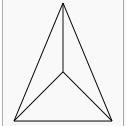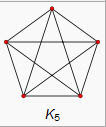Now a spanning Tree is a graph with N vertices but no complete circuits. You can get from any vertex to any other, but only by one path. Here is an example of a tree with six labeled vertices.Finally, we can give the question... For a complete graph of 3 vertices, 4 vertices, or n vertices, how many different spanning trees are possible?

The amazing result is that the two problems seem to be exactly the same, at least they produce the same sequence. The number of solutions for the parking problem is 1, 3, 16, 125, ... (N+1)(n-1)... For the spanning trees, if we start with a graph of 2 points, there is only one tree. For three points there can be three different trees, and for four... yeah..16 . The image below shows all 16 of them as depicted by Wolfram Mathworld.. the sequence is the same but the index is off by one, so we get 1, 3, 16, 125, ... (n)(n-2)... Ok, here is the real question for this blog... why are they the same...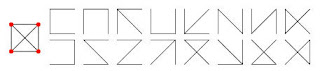Ok, one more curiously beautiful detail about this problem.... the probability of success for the n parking cars at the top has a limit of e/(n+1) as n approaches infinity. I love how often e pops up in these "sorting" type problems.

## Friday, 25 December 2009

### Merry Christmas

Stole this off John's Post at The Endeavour, Well worth repeating

## Wednesday, 23 December 2009

### Exploring an Isoperimetric Theme

Recently we have been doing maxima/minima applications in calculus. By the time they get her most of them know that when they maximize the area of a rectangle with perimeter constraints, they get a square. In Pre-calc we do some basic problems with 2 adjacent pens, a single pen against a wall or river, and all the similar cases. I have written about the generalizations of some of these here, and here. The problem of two adjacent congruent pens is (or should be) easy for a calculus or precalc student.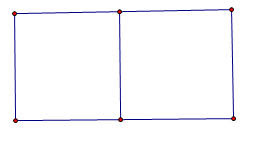It turns out that the maximum occurs when the total width is one-fourth the perimeter and the height is one-sixth the perimeter, for a maximum area of one twenty-fourth of the perimeter value (in square units, of course). If you extend that to even more congruent rectangular pens placed end to end it turns out that the width stays the same?

If you extend this, as many teachers do, to a shape like this one, it starts to get interesting to the more observant student.The height is now one-eighth of the total , and the width is one-sixth of the perimeter. Even slow students can figure out that the area of the total turns out to be one forty-eighth of the value of the perimeter. Some will even generalize the area of an array of mxn congruent pens to be the perimeter squared divided by [4(m+1)(n+1))].... but it seems to take forever for some one to finally realize that in all these problems, whether there was one rectangle, or one with one side adjacent to a river or barn, or rows of rectangles... there is one invariant...

Theorem one for rectangular arrays of congruent rectangular pens "The amount of fence going right to left is the same as the amount going up and down." If you want to maximize a rectangular array with five rows of three pens in each row, we will need 24 lines of fence, four going vertically, six going horizontally. Use half the fencing to make the four vertical fences, and the other half to make the other six going horizontally. (Prove..... dear reader) ..

I always thought this fifty-fifty use of fencing was a cute mathematical "teacher trick" but I have recently come to believe there is something deeper at work. I played around recently with this problem but loosened the constraints on the pens to be just equal area rectangles rather than congruent. This offered some nice areas to explore. Which would be better, three congruent pens in a row, or two pens with a third beneath... like so.The three adjacent pens turn out to have a max area when the width was p/4 (where p is the perimeter) and the height was p/8, giving a maximum area of p2/32... and as before, that mean that half the perimeter was used for the vertical sections, and half for the horizontal sections..and for the three non-congruent sections with equal area the width was p/6, and the length was 3P/16, giving a max area of....wait, that's also p2/32. (Ok, I hadn't expected that)... but when I checked the dimensions, it turns out that the horizontal sections use up exactly half the perimeter, and the vertical sections the other half in both cases.... hmmm...

Ok, but every kid knows that the area is maximized when the rectangle is closest to a square... so what if we just put three squares arranged as shown below and didn't require that the whole assembly forms a rectangle... I thought that would probably be even more area..It turns out that it isn't, The equal distribution of left and right still works out, but the total area is only 3p2/100 or about 96% of the other shapes. (ok, I wasn't ready for that)...

So, I decided to move up to five pens... I passed over four absolutely sure that the division into four equal squares in a two by two array would be the most efficient, but five offered several variations, five in a row; two rows of two with a single in the third row, or three in one row and two in another... The five congruent squares in a row gave a max area of p2/48, but the set up with three pens in one row and two in another gave a slightly larger area of 5p2/216 or p2/43.2. The 2,2,1 set up (shown below) gave not quite the same area..This set up gave a max area of p2/44.8.

From all of this I have a formed a totally unproven conjecture about the maximum area when n equal area rectangular pens are set out in a rectangular boundary with a given amount of fencing.
The Theorem One above for congruent rectangles seems to be a stronger property than I suspected, and is applicable to all equal area pens in a rectangular boundary, And the conjecture is that when the number of pens, n, is between x2-x and x2+x, the maximum area will be found when there are x-rows of pens and all of the pens have either x-1, x, or x+1 pens in the row.

The number of pens in any row will never differ by more than one from any other row. For values of n that are perfect squares or pronic (product of consecutive integers), the number of pens in each row will be the same. When n > x2 there will n-x2 rows that have x+1 pens, and the remaining rows will all have x pens. Similarly, if n is less than x2 there will be x2 - n rows that have x-1 pens and the remaining rows will have x pens each. As an example, if n= 23, then x=5 since 52-5 is less than 23<52+5. And for 23 pens there will be five rows with x=five pens in three of them, and x-1=four pens in two of them.

I also have discovered that the theorem about equal lengths of fencing horizontally and vertically seems to be more important as a "rule of thumb" for maximizing than is equal sides. For example if five squares are set out as efficiently as possible (four in a square with one attached to a side), the area will be p2 / 45 with 8/15 of the perimeter going one way(say horizontally) and 7/15 going the other. If we change the squares to rectangles with each having a height of p/16 and a width of p/14 the area is p2/44.8.. an improvement of about 1/2 %....
Even if you go to six pens (where there is no lost perimeter enclosing the odd pen), putting two rows of three squares gave me an area of P2 / (48 1/3) but using the equal division of fencing gives the very slightly improved maximum of p2/48.

I can't explain why, but the equal division of fencing theorem is stronger than I first suspected, and if someone out there can shed more light on it than my feeble exploration, please do.

I haven't had time to expand the formula for the max area of all n when a rectangle is divided into congruent area rectangles, (not too difficult, I think).. but for square or pronic numbers of pens, it seems the max area will always be p2/(4 (x+1)(y+1)) where xy=n. As a limit as n approaches infinity, it seems the max area for rectangles divided into n equal area rectangles approaches### Learning Styles Theory BOGUS?

Hat tip to Steve Phelps who put me on to this article... Matching Teaching Style to Learning Style May Not Help Students.

Some clips from the article:

"There is no strong scientific evidence to support the "matching" idea, they contend in a paper published this week in Psychological Science in the Public Interest. And there is absolutely no reason for professors to adopt it in the classroom."

"Lots of people are selling tests and programs for customizing education that completely lack the kind of experimental evidence that you would expect for a drug," Mr. Pashler says. "Now maybe the FDA model isn't always appropriate for education—but that's a conversation we need to have."

I think the report trys to be balanced... 'Advocates of learning styles respond that Mr. Pashler is the one who lacks evidence. Robert J. Sternberg, dean of arts and sciences at Tufts University and a psychologist who has done a lot of work on learning styles, says in an e-mail message to The Chronicle that the researchers did not fully survey the scholarly literature, and thus "come across looking either biased about or largely ignorant of the field."'

Read and draw your own conclusions...but the one line that resonated the most with me is this one...."For a given lesson, one instructional technique turns out to be optimal for all groups of students, even though students with certain learning styles may not love that technique." Lesson (for this teacher) Let the lesson you are teaching drive the instructional strategy.

## Sunday, 20 December 2009

### Puzzle Master Scott Kim

For all the kids who fiddle around with the puzzles in my classroom, and those who wish they could...

## Saturday, 19 December 2009

Mathematics of origami..... flapping birds and space telescopes, and geometry like you OUGHT to see it..... For all my students who think geometry is disposable

## Friday, 18 December 2009

### Exponential growthMy Senior math kids are studying exponential curves now, and their inverses, logarithmic curves... it seems hard to get across how fast they grow, but then you flick on The Endeavor, and he has this cartoon from Abstruse Goose... Sometimes a cartoon is the best teaching tool...
Thanks John...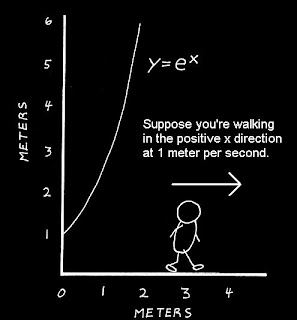## Wednesday, 16 December 2009

Recently someone on the Calculus EDG asked about the value of. I sent a link to some work I had done a while ago exploring the same idea, and extending to finding the value of. I have picked out some parts below, but you can see the rest at this link. This is a very old Word Document so give it some time to load. Hopefully it is worth while. Dave Renfro then sent me a copy of some papers about the topic, including this one from a 1935 American Mathematical Monthly.

When you take the iterated square root of a number, such as $x = \sqrt{n+ \sqrt{n+ ...}}$ and then square both sides, you get $x^2 = x + n$. This means that we can find solutions using basic quadratic solution approaches, and then find solutions that produce integer values of x. The positive solution becomes $\frac{\sqrt{4n+1}+1}{2}$

One of the nice things I discovered was that the iterated square roots of 2 was not the only number that gave an integer answer. In fact, 2, 6, 12, 20, 30.... all were equal to integer values... This sequence is the pronic or oblong numbers, which are twice the triangular numbers. These numbers can be expressed as (n)(n+1) or n,sup>2 + n. It took me a moment to realize why they are the ones that would work. These are numbers that, when multiplied by four and increased by 1, become perfect squares, $4 (n^2+n)+ 1 = 4n^2 + 4n+1 = (2n+1)^2$. And the square root, being an odd (2n+1) number so that when 1 is added, we get a number divisible by 2.

It seems, according to the Herschfeld article, that the problem was a common topic in the Columbia classes of Dr. Edward Kasner. Kasner, of course, is known for his part in the creation of the term "googol" for 10^100. If your interested in any of these topics, check either or both the links above .

I had not yet tried to consider the roots of the cube root of (a+cube root(a+ .... etc)) and so I wanted to take a shot.. By the same process I had used before, the value would be the solution to x^3-x-n=0 .

If the iterated value was 1, the value approaches about x=~1.32472. For n=2 the value is x=~1.52138. By the time we get to n=6, we get x=2. The actual solution for any n isOK... that really isn't very much fun to play with, but after some experimenting, I came up with the fact that the following sequence of numbers produced integer values when iterated; 6, 24, 120, 210... ; or perhaps it is more revealing to write them a different way (1*2*3) , (2*3*4), (3*4*5)... so they were sort of the three dimensional pronic numbers, the products of three consecutive integers.
I could not manipulate the above equation to make it clear that these were the only values as I had with the quadratic, but it got me thinking, what if I did fourth roots ?
Extending the solutions for square and cube roots, I tried 1*2*3*4 = 24.... but the solution of $n^4 - n- 24=0$ was NOT 2; in fact, it was about 2.1617???
Exploring I found that n=2 was a solution to $n^4 - n- 14=0$. And n=3 was a solution when the constant was 78. The sequence is 14, 78, 252, 620, 1290,... These values follow the form n*(n-1)*(n^2+n+1)
I realized, somewhat belatedly, that you could generate these sequences by simply using nk - n for integer values of k, and factoring the same would give you the simplified form of the expression.
After struggling with solving n^3 - n -k=0 I realized that I could just start with values of n, and find out what k came out to be. A very late "aha" moment. So for n=2, 23 - 2 = ??? and six pops out like Alg I.

There is a familiar quotation about forests and trees that seems to apply here, but it came to me somewhat late.

But sometimes, that's how my mind works... do it the hard way first.

## Tuesday, 15 December 2009

### Folding a cube???

Found this at Math Recreations by Dan MacKinnon. Fold a cube into..well,some interesting stuff.
The origninal work comes from Eric Demaineclick here, then wait, it takes a minute to animate...but well worth it.

## Monday, 14 December 2009

John D. Cook over at the Endeavor always has great stuff, and today his blog led me on a trail to the video below.... only one word...weird... There is no trick, it's just between you and your lying eyes...

## Sunday, 13 December 2009

### Fox Math>

Found this on MediaMatters, where they say it appeared on the December 4 edition of Fox & Friends. You do the math.Kind of goes with their recent Pie-Chart "new-math" approach.

## Saturday, 12 December 2009

### Problem with Unexpected ResultHere is a quick problem with a result which surprised me...

Graph a semi-circle with the center at the origin ... then graph y=2x... The point of intersection is the right angle of a right triangle whose hypotenuse is the diameter. Find the ratio of the legs...

## Wednesday, 9 December 2009

### Fun With Parabolas in Calculus

Ok, two quick mental problems. Some folks will see one as somewhat easier than the other, but ...well heck, read on...

Problem One. A secant cuts the parabola y= x2 at x= -1 and x=1. Find the area of the region between the secant and the parabola..Problem Two. A secant cuts the parabola y= x2 at x= 1+sqrt(5) and x=3+sqrt(5) . Find the area of the region between the secant and the parabola..

Ok, if two seems to hard, try this middle of the road alternate:

A secant cuts the parabola y= x2 at x= 0 and x=2. Find the area of the region between the secant and the parabola..I still find it fascinating each year as I introduce my calculus class to this idea that the answers are all the same. I lead them eventually to conclude that the area between the secant and x2 depends only on the difference between the two x-values.

I was recently reminded of this little parabola property when the very kind Dave Renfro put me on to an article in the December Monthly from the MAA by Bettina and Tom Richmond entitled "How to Recognize a Parabola." Among a dozen really nice properties of parabolas, the first one was this little area relation. They included several that I had used recently in my blog about tangents to a parabola and circle. They also had another theorem closly related to these problems in their eleventh example which says (in my less rigorous language) that the area of the region between the secant and a parabola is 2/3 the area of the parallelogram that inscribes that region. The two ideas together mean that the perpendicualr distance between the secant and the tangent parallel to it when multiplied by the length of the secant is a constant depending only on the difference in the x values also. ... In the region cut off by the horizontal secant the distance from the secant to the vertex is 1 and the length of the secant is 2. Two-thirds their product gives the area of 4/3 sq units. In the region cut off by the secant from x=0 to x=2 the secant has a length of sqrt(20). The tangent parallel to the secant will be at x=1 (that would be Theorem 3 in the Richmonds' paper, "the average of the slopes at the endpoints is the slope at the midpoint" ). The perpendicular distance from the tangent point to the secant line is 1/sqrt(5) , and the product of these two distances is again 2.

The relation between the two above has started me wondering about their tenth theorem, which I had not observed before, and a possible generalization of the theorem. The theorem said that if you took the section formed by a horizontal secant and the parabola, and revolved it around the y-axis, the volume inside the bowl and the volume remaining from a cylinder when the bowl is cut out are the same. Ok... but driving to work I got to wondering, If we took and oblique secant, and rotated the area between the secant and the parabola about the line perpendicular to the secant passing through the point where the tangent would be parallel, would that also have the same volume? (I keep thinking it would, will leave the exercise prove or disprove to the reader).

By the time I get around to writing this, I'm thinking it is NOT the same as the horizontal case... but I keep thinking when I get to it there will be something pretty hiding here...

## Thursday, 3 December 2009

### The Shoestring ExplainedOne of my students got too little sleep last night because he kept trying to figure out why the shoelace algorithm worked. One of those really bright kids who transferred in and just hasn't had an education up to his potential. He has never been introduced to vectors, so after a very cursory overview of the idea of vectors and cross products, I showed him the following as a way of "explanation". He had seen determinants in Alg II but never used to find area.If we take the the set of vertices and add one more point at the origin, then we can draw vectors from the origin to each pair of consecutive vertices. In the first image we have drawn the vetors [1,1] and then [5,2], and we show the triangle area formed between the two vectors. The Determinant of the two vectors will give us twice this area. If we imagine the area being swept out by the first vector rotating until it reaches the second, a rotation of a positive direction will give us a positive value for the determinant, and a negative rotation will give us a negative value. Since we started at (1,1) our first area is negative, and equal toor -3/2.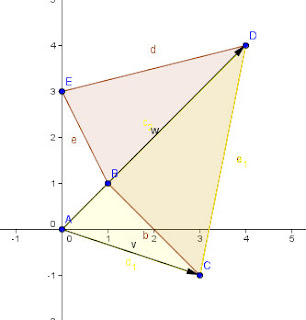As we proceed in order from vertex to vertex, the vector triangles will add and subtract areas as it sweeps around the polygon until it gets back to the starting point. The Vectors from [5,2] ot [4,4] add back the area just subtracted in the first pair of vectors, and also the area inside the polygon formed by triangle BCD (this is just by chance because vectors AB and AD have a common slope)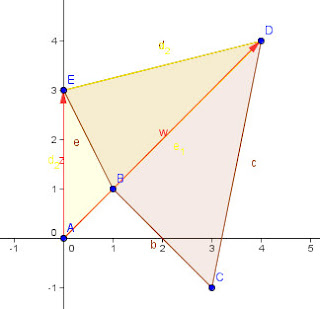The next pair of vectors add the rest of the polygon, but also some excess in the amount of triangle ABE. This excess is removed when we sweep back to the original point A and so we have the final area of the polygon.If we had traversed our way around the vertices in the reverse order, the determinants would have all had their signs reversed and we would have a total which was the negative of the actual area. Hope that is pretty clear, there was a lot more armwaving when I was doing this at the board.

## Wednesday, 2 December 2009

### The Shoelace formula and Formulas for ShoelacesThe shoelace formula is an algorithm for finding the area of a polygon in the plane when the coordinates (x,y) are known.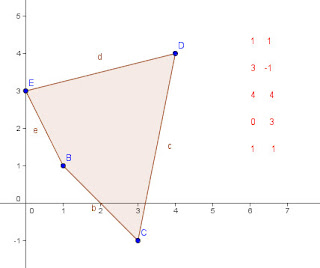For example if the coordinates of a quadrilateral are given as (1,1); (3, -1); (4,4); and (0,3) then the area can be calculated by putting these in two columns (or rows as shown below) and multiplying along diagonals as shown (notice that the first coordinate pair are repeated at the end for ease of computation).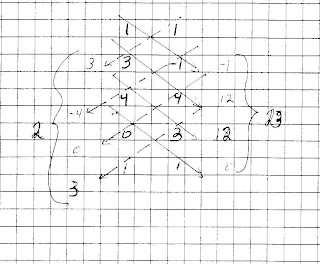The diagonal products to each side are summed, then the absolute value of the difference of the two sums is multiplied by 1/2 to give the area. In this case the right products total to 23, the left to 2, for a difference of 21, so the area is one-half of that, or 10.5 square units. The points have to be taken in order around the polygon in either clockwise or counter-clockwise order using each number once as the first pair. It is the criss-cross appearance of the diagonals that draws the "shoestring" name to the apporach.

The shoelace formula is just a simple diagrammatic extension of an earlier determinant method sometimes (perhaps seldom?) called the Surveyor's formula (not to be confused with the Ptolemaic Egyptian method of the same name for finding areas of quadrilaterals), which does the same computation with a sequence of determinants, like this: Note that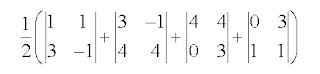This gives ½(-4+16+12-3) which is again the 10.5 sq units we had before. The calculation of each determinant constitute one product from each side of the shoelace algorithm and calculates their difference. (the sum of the differences is the same as the difference of the sums).

Additional illustrations and worked examples can be found at this file by Charles L. Hamberg and Ronald Vavrinek, from the Illinois Mathematics and Science Academy. It also includes a program you can use on your ti-84 or other programmable calculator.

While we are talking about shoelaces, they also showed up in an interesting book by Australian Mathematician Burkard Polster. In "The Shoelace Book", Polster examines various approaches to methods of lacing a pair of shoes, and "mathematizes" lacings formally enough to enumerate the possible lacing paths.. For a shoe with six eyelets on each side there turns out to be 43,200 different paths for a shoelace to pass through every eyelet, even with the added condition that each eyelet must contribute to the essential purpose of pulling the two halves of the shoe together..... go ahead, take a minute and check...I'll wait... dum de dumm..de dumm (all done?) OK.. If that was more difficult than you might have expected, you can find a good link that gives a glimpse of the work at Google Books where he describes lacings by such names as Crisscross, zigzag, bowtie, devil, angel, and star... I'm thinking of switching to the bowtie lacing myself, not just because it is efficient, but I like the look.
You can also order the book from Amazon

Speaking of strings (ok, that's a stretch from the "shoestring" idea) this is blog number 300 on Blogger.... I think I'm a veteran...

## Tuesday, 1 December 2009

My version of a math joke I came across recently.

How many mathematicians does it take to change a light bulb?

Answer: None, it may take many mathematicians to prove (or disprove) that a light bulb may be changed, but once that is established, .....the number of mathematicians it takes will be left as an exercise for the reader.

## Saturday, 28 November 2009

### The Mathematics of RiversDave Richeson, who writes the Division by Zero blog is an Associate Professor of Mathematics at Dickinson College and also the author of a really good math book, " Euler's Gem: The polyhedron formula and the birth of topology" from Princeton University Press.

He also just introduced me to a word I didn't know, potamology, from the Greek ποταμός, river. The word is the technical name for the study of Rivers. Incredibly, there is some really cool math and statistics involved in the study of rivers. For one thing, they are sort of fractal, or as Dave explained it, " the size of a river cannot be determined by its shape on a map. In particular, if you looked at an aerial snapshot of a meandering river, you would not be able to tell whether it is the Amazon or a small neighborhood stream!"

Dave goes on to relate how a the distance between two meanders in a river are related to its width. If we let the width be w, and lamda be the distance between the beginning and ending of one not-quite-sinusoidal period of the meander, then lamda = 11w.
For stats kids, he also posts a regression plot of the actual ratio between meander length and channel width....stats in action baby.

Go to Dave's site and read the whole thing.... he has cool pictures for examples also, including the one above.

## Wednesday, 25 November 2009

### A Simple Geometry ExplorationIt is a simple geometry question... if you hold the perimeter of a convex polynomial constant, what are the possible limits on the sum of the diagonals, in particular, what is the maximum. I realized I was pretty unsure about the solution, even for a quadrilateral, the simplest case. I thought about a rectangle, and realized that the diagonals get longer as the sides become less equal. If we assume the perimeter is p, then any two sides of the rectangle would be x, and p/x-2. The two equal diagonals would each be the square root of (x^2 + (p/2 -x)^2)) which is largest when the sum of the squares is largest. But the sum is 2x^2 - px + p^2/4 . This is a positive quadratic, and so it is greatest at the ends of its domain, and smallest at the vertex (p/4)... a square has the smallest sum of the diagonals of any rectangle. This is easy to confirm if you take a 4x4 square which has two diagonals of 4 sqrt(2) for a total of 8 sqrt(2) (more or less 11.314), but if we put make it more oblong, say a 7x1 rectangle, the diagonals are each sqrt(50) or 5 sqrt(2) for a total of 10 sqrt(2) (a larger 14.142)... if we extend this to the limiting behavior, we see that each of the diagonals would be close to p/2 so the total sum of the diagonals would approach the perimeter as a limit.. Trying different shapes led me to think (but not prove) that p is probably the limit for a quadrilateral.

But what happens if we let the number of sides go beyond four.... I had no idea how to approach the problems except to experiment. I began by drawing five points on a circle and taking the ratio of the sum of the diagonals to the perimeter. I quickly realized that if I moved two of the points close together on one place and three others near the opposite side of the circle the ratio approached two.. the diagonals were nearly twice the perimeter. This made sense, three of the edges would be nearly zero, and two would be almost the length of the diagonal of the circle, so the total perimeter would approach 2d. And what of the diagonals? Well, there would be four diagonals that went from the two points on one side to the opposite three points that would each be approximately the length of the diagonal, for a total of 4d.

Would this be better with four on one side and one on the other? The perimeter would be the same, but there would only be two long diagonals. It seems that splitting the points up evenly increased the total sum of the diagonals. It seems the diagonals would sum to 2p in the limit. I couldn't imagine exceeding this (correct me if I have failed to visualize something here) for a pentagon...

So, what about a hexagon......? six edges, and 9 diagonals.. . Using our previous insights, we could try putting 3 points close together on opposite sides of the circle, This would mean that would mean that the total perimeter was again approaching 2d, but there would be 7 diagonals which were also approaching the limit of a diameter. The other two diagonals would approach zero, and the total sum of the diagonals would be 7d, for a ratio of 3.5......

Hmmmmm could I see a pattern here? for n=4, the ratio of diagonals to perimeter was 1, for n=5 sides, the ratio was 2, and at n=6 the sum would be 3.5. Well, for a four sided figure, there were two diagonals (and essentially all the perimeter was in two sides, 2/2 =1 ) . For a pentagon, there were 5 diagonals, but one of them went to essentially zero... the ratio was 4/2 or 2. With a hexagon there were 9 -2 long diagonals, so a ratio of 7/2. Could I extend this?

If we went to seven sides, there would be 7 choose 2 - 7 diagonals, or 14 of them. We would put four on one side and three on the other. By my count there woud be 10 long diagonals and four that diminished to zero (one on the end with three points connecting the outside two, and three on the end with four points) so the ratio would grow to 5 times the perimeter

lets tabulate what we know (or think we know)

n-sides... total diag.. short diag...long diag.. ratio --- change
4----------2----=======--0-------------2------------2/2=1---------0
5------------5------------1--------------4-----------4/2=2--------1
6------------9-------------2-------------7-------------7/2--------1.5
7-----------14-------------4------------10------------10/2=5------1.5
8-----------20------------6-------------14-----------14/2=7 -----2
9-----------27------------9 ------------18-----------18/2=9-------2
10----------35-----------12-------------23----------23/2=11.5----2.5

Ok, the recursive pattern seems to be r(n+1) = r(n) + floor(n/2)/2... I think I did that right....

and it is late, so I will let you write the explicit function...I'm just thankful that the holiday is at hand. I'm off to London with my sweetheart for the weekend to see a west-end show and have a good Japanese meal... and hold hands and walk through the neighborhood markets... hope you have a great holiday too.

## Sunday, 22 November 2009

### Scientists Say the Stupidest Things

I was in Cambridge recently with my beautiful sweetheart, and we were browsing through Oxfam when I came across the book, Foolish Words, The most stupid words ever spoken by Laura Ward. It is gut wrenchingly funny in places (and also terribly sad at the same time), but I especially treasured some of the bold predictions about the future from people who would be expected to have a better than average insight into the topic. It reminds me of the wisdom of a quote on my classroom wall, "Never miss a good opportunity to shut-up."

Here are a few of them:
"The energy produced by the breaking down of the atom is a very poor kind of thing. Anyone who looks for a source of power in the transformation of the atom is talking moonshine."
Lord Ernest Rutherford after splitting the Atom in 1933

Einstein had said only a year earlier, "There is not the slightest indication that atomic energy will ever be attainable. It would mean that the atom would have to be shattered at will."

Here is the Astronomer Royal of Great Britain, Sir Richard Woolley, in 1957, "I cannot see any nation or combination of nations producing the money necessary to put a satellite in outer space or to circumnavigate the moon." He was actually expanding earlier remarks (not in the book) when, on his appointment as Astronomer Royal, he reiterated his long-held view that 'space travel is utter bilge'. Speaking to Time in 1956, Woolley noted "It's utter bilge. I don't think anybody will ever put up enough money to do such a thing . . . What good would it do us? If we spent the same amount of money on preparing first-class astronomical equipment we would learn much more about the universe . . . It is all rather rot". Woolley's protestations came just one year prior to the launch of Sputnik, five years before launch of the Apollo Program, and thirteen years before the first landing on the moon. (from Wikipedia)

Thomas Edison in 1928, "I have determined that there is no market for talking pictures."

German Physicist/chemist Johann Poggendorf proudly announced, "It is impossible to transmit speech electrically, the 'telephone' is as mythical as the unicorn."

Simon Newcomb, one of the most brilliant men of his period, a polymath and perhaps the first discoverer of Benford's law, among other things; stated boldly, "Aerial flight is one of that class of problems with which man cannot cope", 1903. (for my students who know so little history they make me cry, it was in December 17, 1903 that the Wright Brothers made their famous flight at Kitty Hawk.)

In March of 1949 an article in Popular Mechanics wrote, "..the Eniac is equipped with 18,000 vacuum tubes and weighs 30 tons, computers in the future may have only 1000 vacuum tubes and weigh only 1.6 tons." (or perhaps, 1.6 pounds?)

Lord Kelvin, President of the Royal Society, and so esteemed that he was buried in Westminster Abbey next to Isaac Newton, said, "X-rays will prove to be a hoax" and is also known to have said, "Heavier-than-air flying machines are impossible" in 1895. Then he went on to doom another budding invention, "Radio has no future." Perhaps he had anticipated the birth of television.

Lee DeForest was an inventor whose work ushered in the electronic age. The inventor of the tube which made audio amplification possible and bringing sound to the motion picture went on to proclaim, "While theoretically and technically television my be possible, commercially and financially it is an impossibility."

Finally, a quote from Dr. William Clark, president of the Arthritis Foundation in 1966, "We just won't have arthritis in the year 2000." (I have it on good authority from my aching shoulder that it is back by 2009).

I guess I leave the last word to that Great Statesman, George Bush, who explained it all as an educational problem when he stated in 2000, "What's not fine is, rarely is the question asked: Is our children learning?".

## Friday, 20 November 2009

### What Can You Say About a Polynomial with All Coefficients Equal

My lunch math kids and I came across a problem about a function with all its coefficients equal (hereafter called PWEC, polynomials with equal coefficients). I had to admit to them that I had never thought about such a function, so we developed an interactive graph on geogebra and played around with it, looking for what we might say about such a function.. So here is your chance to show how clever you are...

I took a couple of the kids observations about PWEC functions and intentionally violate at least one of them in each graph. Each of the functions is NOT a function with all coefficients equal; and your task is to say WHY? Some have more than one reason...

Game on.. here we go:Can you get it? If not, scroll down a little to find at least one reason...
a
a
a
a
a
a
a

Ok, observation number one, Joe S picked up on this one right away... Every odd powered PWEC will have a zero at x=-1... easy to confirm because the alternating powers will be negative and positive. Descarte's rule of signs proves that there can be no real zeros that are positive. My experience is that it always has just one; the one at x=-1.

Did you get it?? Well, how about another...a
a
a
a
a
a

This time you can't get much from Descarte, but it seems from observation that an even PWEC can never have a zero. I played with this a little and haven't succeeded in proving it analytically for anything but a few simple polynomials.. any offers?

Ok..just one more... another even function... what do you think...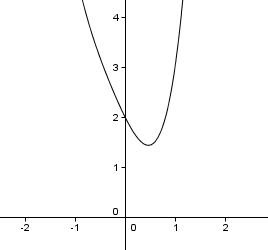OK, no easy stuff on this one... what do you think?
a
a
a
a
a
a
a

a
a
a
a
This time there are no tricks with the zeros... Ok, maybe one more.... notice that the horizontal tangent occurs with x>0...but that is a no-no as well. The derivative will also have all positive coefficients, so by Descarte's rule of signs again, it can not have a zero when x>0.

I admit I had never thought of any of these things, and noticed them all by working with interactive graphs on Geogebra.. one reason I am thankful for the power of modern computing... I know more math because of the technology available.. and I want my kids to see that it can be more than an easy way to get the answer, it can help you learn more about the ideas of mathematics.

## Monday, 16 November 2009

### More on the Common Tangent Problem

I mentioned in my last blog that I had been exploring the relationships around the problem of a common tangent to a circle and a parabola with a vertex at the center of the circle. To make notation easier, I have assumed that the center and vertex are at the origin and the axis of symmetry is along the x-axis. I came across a couple of unexpected (to me) relationships, and so I thought I would present them in the form of a problem, with the answer further below.

If I understand the relationship (no guarantees) then there is a unique solution to each point.

Problem: A circle centered at the origin with a radius of r shares common tangents with a parabola y2 = ax. If the tangent contains the point (p,q) on the circle; find the coordinates of the tangent point (s,t) on the parabola?

a
b
c
d
e
f
g
h
i
j
k
l
1
2
3

Ok.. here is the things I noticed...

a) For the common tangent to a circle x2+y2=r2 at (p,q) and a parabola, y2= ax at (s,t) the product of the x-coordinates of the tangent points is equal to r2... ie ps=r2

b) and the product of the y-coordinates of the two tangent points is twice r2, or qt=2r2

c) the x-intercept of the common tangent is the negative of the x-coordinate of the tangent point on the parabola.

My students like solutions with numbers as examples, so here we go... if the tangent point on the circle is at (-3,4) then the tangent line will be the equation -3x+4y=25
The x- intercept of the tangent line is at -25/3, so the value of the x-coordinate of the tangent point on the parabola is at 25/3.

The y-intercept of the tangent line is at 25/4, and so the y-coordinate of the tangent point on the parabola is 25/2.

Now that we know x and y, we can find a, since y2 = ax; we can write (25/2)2 = a(25/3) . Then a must equal 75/4...
Checking children, we know the slope of the tangent to y2 = ax will be a/2y which is (75/4) divided by (25).... but that is 3/4, and of we know that the tangent is perpendicular to the radius going from (0,0) to (-3, 4) which agrees with m=3/4 .

The real challenge now, if you are up to it, ....Assume the circle has a radius of r (pick your favorite value), and the parabola is y2 = ax (pick your favorite a) and now, find the equation of the tangent line, and the points on each where the tangent point falls.

## Tuesday, 10 November 2009

### Why Didn't I know This Already?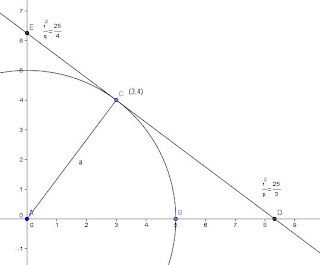I was playing with a problem that Dave Renfro sent to the AP Calculus EDG and got curious about something else, and stumbled upon a simple relationship about tangents to circles that I never knew (or don't remember??? )

The problem Dave sent was about finding the common tangent to a circle centered on the origin and a parabola with a vertex at the origin. I wandered over to playing around with a general solution, and along the way I remembered a cool idea that was known way back to Apollonius, I think. If you have a parabola centered at the origin, like y=ax2, and you draw a tangent to some point, call it (p,q), then the y-intercept of that tangent line will be at (0,-q)... no matter what the value of a is. And it seems clear that a straight line that goes from (p,q) to (0,-q) must have an x-intercept at (p/2,0)

For example, if you used y=x2 at the point (2,4) the tangent would have an equation of y-4 = 4(x-2) and when x= 0, the y-intercept is at (0,-4). If instead you pick y= 3x2, when x=2, y= 12. The equation of the tangent will by y-12 = 12(x-2) and the y-intercept will be at (0,-12), the x-intercept at (1,0)in both cases.

It occurred to me that probably all the conics might have simple relationships that would predict intercepts of the tangent. So I started with a circle.... say x2+y2= r2. What happens if we pick a point (p,q)? As I wrote in a recent blog, an easy way to find the tangent to a conic is by the use of polars. If we want the tangent to a circle at a point (p,q) we need only to replace one x and one y in the equation with the values p and q. In the case of x2+y2= r2, the tangent through the point (p,q) would be px+qy=r2. Pretty easy (so why had I played with this all these years and never noticed that the x-intercept would be r2/p, and the y-intercept would be r2/q. So why had I never noticed this relationship??? (don't answer, the truth hurts)

So a simple hyperbola or ellipse could be done the same way... tooooo easy...

## Sunday, 8 November 2009

### Now Can You See the Solution???

A short while ago I pointed out that kids could "see" the complex solutions of a quadratic in a relationship with the vertex and the leading coefficient. You can make that visualization a little more evident with a couple of graphs. The students could already knew that if you graph a quadratic with real roots, then you can "see" them in the x-interceptsThe graph shown is y= (x-2)2 - 16 = x2-4x -12. One of the things I try to get them to see is that if you break the quadratic formula into two parts, it will give you a better sense of what is happening. In the example above the quadratic formula gives $x=\frac{4\pm \sqrt{64}}{2} = \frac{4}{2} \pm \frac{8}{2}$.

I want them to see that if they break it into two fractions, the first part gives the axis of symmetry, 2, and the second gives the distance from the axis to the two real solutions, 4. If you make the a coefficient larger, the curve will get to the x-axis sooner, and the two solutions will not be as far apart. The next image shows a quadratic with the same vertex, (2,-16) with a leading coefficient of two.The solution then, will cross the x-axis at a distance from the axis of symmetry which is now the square root of 8 (16/2) instead of the square root of 16. As a quadratic moves c units to either side of the axis of symmetry, the quadratic will change its y-value by an amount equal to Ac<2. Setting this equal to 16 (the distance of the vertex below the x-axis) we find the distance from the axis of symmetry to the roots. But if we look at a graph of a quadratic with complex roots, we don't see any such distance to each side of the axis of symmetry....but we can... using a method that may have first been suggested in Howard F. Fehr, "Graphical Representation of Complex Roots," 'Multi-Sensory Aids in the Teaching of Mathematics', 'Eighteenth Yearbook of the National Council of Teachers of mathematics'  pp. 130-138. George A. Yanosik, "Graphical Solutions for Complex Roots of Quadratics, Cubics, and Quartics," 'National Mathematics Magazine', 17 [Jan. 1943], pp. 147-150.] The next graph shows a graph of a quadratic with the vertex at (3,5) and a leading coefficient of positive 2, which has no real roots.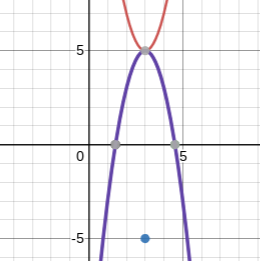But if we graph the quadratic with the same vertex and a leading coefficient of the opposite sign, it will cross the x-axis at a distance away from the axis that is the same as the roots of $y=2 (x-3)^2 -5$ . These distances imaginary coefficients of the complex solutions. AND... If you rotate the entire coordinate plane by 90o, the two points will also be the endpoints of the Argand diagram of the two solutions. I'm hoping that if a kid can fit all this together, they will begin to understand quadratic equations, their graphs, and solutions a little more. If not, we can try solving them by Newton's approach with log scales... but more about that some other blog.

## Friday, 6 November 2009

### And Number 1000 Should Be

For a long time I've been studying the etymology and history of math words (and other things I thought were fun) and recording what I have found at my MathWords web page. A very amateur contribution, but a labor of love that has found its way into a few nice corners of math study at different levels... And today I added my 999th term... and now the quandary begins; What should be number 1000 to celebrate the moment. (to be honest, when I had 100, I really figured I pretty much had the landscape covered... wow... )
I would like it to somehow relate to the number itself, but I have used up all the ones I know.. SOOOOOooooo dear reader, have you any suggestions? Something profound. Something fitting for the moment of "chilioi-ness" (the root which gave us kilo, except the ending, I made that part up).

The world awaits.... History may yet hold a place for you as the one who first suggested this momentus term... Full credit in print, my gratitude, (and almost certainly a pot-load of temporary fame) will be yours.

### Congruent Numbers?

Ok, I'm as old as dirt, and I never heard of congruent numbers, which is bad since they sort of go back to the Dark Ages, and Fibonacci wrote about them, and Fermat DID have room in the margin for a proof about them... but a recent blog at at Bit-Player about it after a recent news release from AIM (American Institute of Mathematics) announced that all the congruent numbers up to 1 trillion have been enumerated. Well, job done I guess. The blog is so well written that I am not about to try to replicate all that good work, go read it. If you want more, here is a link from the AIM on the same topic. Enjoy, I certainly did.

## Thursday, 5 November 2009

### An Interesting Counting Problem...

I came across an interesting problem on a video from Shai Simonson about Discrete Math. The question was to count all the possible permutations of a subset drawn from n items.

The count would seem to be direct enough. Just take every possible subset, and count the permutations of that subset. There would only be one subset with n-items and it has n! permutations.. there are n choose k permuations with n items, and they can be permuted in k! ways.. so we could write the whole sum as P(n)=If we enumarate a few we see a pattern: P(0)=1; P(1)=2, P(2) = 5, P(3) = 16, P(4) = 65.... and from that we sense that P(n)= n P(n-1)+1...

Shai had a nice way to show this is true. Here is my illustration of his approach:can be rewritten asand here he does something very clever... he factors n out of each of these terms in the new expression to getwhich is just 1+ P(n-1)...

But what do you do with P(n)= n P(n-1)+1. It doesn't seem to have a close form expression that lets you calculate the nth term directly.

Once more Simonson gets creative. He points out that the derangements of n follow a similar recursive sequence, except that d(n)= n [d(n-1)] + (-1)n .

He points out that this is known to be equal to n! (1/1 - 1/1+ 1/2! - 1/3! + 1/4!... 1/n!)

He then suggests that P(n) could be written as n! (1/1 + 1/1+ 1/2! + 1/3! + 1/4!... 1/n!) which matches the values of all the P(n) I checked, and of course, as n goes to infinity, that is just P(n)= n! e .

Ok, pretty interesting, and in fact, it seems that in all the cases I have tried, the actual value of P(n) is just Int(n! e) . It even works at P(1), and gets closer as n increases.

Try a few, tell me if I overlooked something...

I just thought it was a very pretty math. The comparison to d(n) leading to a really clever limiting value... nice job Sir.

http://www.mathvids.com/subtopic/show/116-combinatorics

## Wednesday, 4 November 2009

### Can You SEE the solution?

It may have been G. H. Hardy who first stated that mathematics is about finding patterns. In his autobiography he writes, "A mathematician, like a painter or a poet, is a maker of patterns. If his patterns are more permanent than theirs, it is because they are made with ideas." It is an idea I often repeat to my students, and to myself when I am trying to present new ideas to them.

We are at that point in the year where we are working with the Fundamental Theorem of Algebra. We have gotten to the point that most of the students can graph an equation, y=f(x) and looking at the graph write the factored form if the roots are all rational. In the vernacular of the kids, the can see the roots. As we began working with complex numbers, one student lamented that they wished you could see the roots as well on a quadratic with non-real solutions. Several others murmured agreement, and it seemed like a teachable moment, so I turned and said, cryptically I hope, "Well, you can, but you have to recognize the pattern." It is hard to believe they thought that there was something simpler than the quadratic formula, but they are more willing to do ten minutes work with a calculator than two minutes with a pencil, and besides, it is pretty, so I was willing to lead them toward it.

I began by setting up some simple problems. graph the equation and find the vertex, then solve by the quadratic formula (never tell them they are actually practicing, we are discovering exciting, not in the book, kind of stuff here).

They do a few:
x2 -2x + 10, with a vertex at (1, 9) had solutions at x= 1 +/- 3i

x2 +4x + 5, with a vertex at (-2,1) had solutions at x= -2 +/- 1i

x2 -6x + 12, with a vertex at (3,3) had solutions at x= 3 +/- isqrt(3)
AHHH, now they had a clue.....

We do a few more, and they seem to be on top of it, but not one has noticed that ALL the problems had a leading coefficient of one... with only a few minutes to go, I gave them
2x2 -4x + 20 (no one notices, it seems, that this is twice the first problem we had done) ... they sketch the graph, trace to x=1 to find the vertex at (1, 18) and hands fly up, eager lips whisper to each other, 3 +/- i sqrt(18), and nods in return assure them they are right... so the teacher springs his trap... pointing, a student responds with certainty... and the teacher tilts his head, and gives him "the eye".... "Did you check?"

A timid young lady offers, "I got an answer of 1 +/- 3i." Heads lean toward each other. What happened. I was sure we had it... and then, an offering of insight... "It's half as much, I mean, its the square root of half of the y part...because of the two in front." But it is almost a question. The bell rings, and no one moves... "Is that it? Tell us."

He turns to the board to hide his smile as he erases.... painfully slowly in their mind..then turns back.... and shrugs... then offers... "Hey, have a great weekend."

## Sunday, 1 November 2009

### Soul Cakes, Halloween Began in Britain??Well, you get old and eventually you learn stuff. I got a note from Charles Wells who said, " The *name* Halloween came from the British. It is the eve of All Saints Day (Hallowmas, November 1) which is celebrated all over Catholic Europe, not just in Britain. November 2 is All Souls Day, meaning the day for sinners as well as saints. That is the Day of the Dead in Mexico."
Later I added, "Thanks, Charles,but given that the Scottish, the English, the Welsh, and the Irish ALL seem to object to being included as "British", I will simply confirm what you have said with a quote from the Online Etymology Dictionary, "c.1745, Scottish shortening of Allhallow-even "Eve of All Saints, last night of October" (1556), the last night of the year in the old Celtic calendar, where it was Old Year's Night, a night for witches. Another pagan holiday given a cursory baptism and sent on its way. Hallowmas "All-saints" is first attested 1389."
I had just seen a BBC show that morning in 2009 and Sting had just released the Soul Cakes song, don't ask why, some things must be left unexplained.
Here is the story of soul cakes and halloween as told by Wikipedia: "A Soul cake is a small round cake which is traditionally made for All Souls' Day to celebrate the dead. The cakes, often simply referred to as souls, were given out to soulers (mainly consisting of children and the poor) who would go from door to door on Hallowmas (new word to me, obviously the eve of All Souls Day) singing and saying prayers for the dead. Each cake eaten would represent a soul being freed from Purgatory. The practice of giving and eating soul cakes is often seen as the origin of modern Trick or Treating." "The tradition of giving Soul Cakes originated in Britain during the Middle Ages, although similar practices for the souls of the dead were found as far south as Italy." "The cakes were usually filled with allspice, nutmeg, cinammon, or other sweet spices, raisins or currants, and later were topped with the mark of a cross. They were traditionally set out with glasses of wine on All Hallows Eve, and on All Saints Day children would go "souling" by calling out: Soul, Soul, a soul cake! I pray thee, good missus, a soul cake! One for Peter, two for Paul, three for Him what made us all! Soul Cake, soul cake, please good missus, a soul cake. An apple, a pear, a plum, or a cherry, anything good thing to make us all merry. One for Peter, one for Paul, & three for Him who made us all. ...lyrics from A Soalin', a holiday song written and performed by Peter, Paul and Mary (1963)."

See, nothing scary here.

## Friday, 30 October 2009

### Counting LinesMy lunchtime math problem solving group was going through some old American Mathematics Competition problems, and we came across one that asked, in slightly more complicated language, "How many lines can be drawn that intersect at least two points in a 3x3 rectangular array?".

Most of the kids who understood the wording got the answer, and when I asked them how, they said they counted... they drew the dots, drew the lines, and counted them...

So I asked, how would you do it if they had a ten-by-ten array?

Counting the number of lines in an nxn array that contain the maximum number of points, n, is pretty straightforward. There are n horizontal lines that contain n points, and another n vertical lines, and two diagonals. For nxn there are 2n+2 lines that contain n points. In the 3x3 case, there are 2(3)+2 = 8 lines that contain exactly three points. So how to count the 2x2.

After a while I decided that there would be a maximum of n choose 2 lines if no two were collinear, so at most, a set of nine points could contain 9 choose 2 = 36 lines. Now each of the lines that had three points, would use up three different lines from this 36.. think of a line with points A, B, and C all collinear. Instead of three lines, they fall on one, so each line of three points would reduce the possible total of 36 by two... and since there are 8 of these lines, we need to eliminate 8x2=16 lines from the 36 to get a total of 20, 8 with three points, 12 more with only two points.

This verified the students solution by counting, but didn't bring us much closer to the general ten x ten case. For instance in a 4x4 there are some lines with four points (10 of them) and some more with three points (a quick look assured me there would be four) and the rest with only two points. By Starting with 16 choose 2 and eliminating duplication as before, I figured out that there would be 48 of the lines with two points.

By the next time we meet, Conner S. had counted up the number of lines with exactly two points by hand up to n=5 (and had a small error in the n=5 case finding only 100 when there are 108... Examining the case for n=5 is influential, but still, we don't get a really good clue of a general pattern. The sequence for the number of total lines from n=2 to n=5 is 6, 20, 62, 140... you might want to try to find the next one.. I couldn't, and the pattern of lines with exactly two points was equally wild... 6, 12, 48, 108??? (For my students, I will show how the counting technique used above to the possible lines given by n choose 2 to find the number of lines with two points. In most cases (such as the five by five) it is not too hard to count the number of lines that contain three, four, or five points.)

I guess I can be ok with my kids not being able to find the pattern, as it seems that the pattern didn't have any simple solution at all, and was eventually extended, it seems, beyond the hand countable cases by computer search. Afterward they did find a summation notation that is way complicated. I finally searched the On-line integer data base and found the actual numbers... don't look if you are still figuring out the pattern for yourself. This triangular array gives the number of lines containing exactly k points in an nxn array.. At the top of the triangle is n=2, k=2, and as you go down each row the n goes up one... so the numbers in the fourth row(for n=5, a 5x5 array), {108, 16, 4, 12} is the number of lines containing 2, 3, 4, and 5 points.Interesting to look down the center at the sequence of 4's, as long as the number of points in the line is more than (n+1)/2 and less than n, there will be four such lines. Predicting the rest of the table as yet is beyond me.. if you see a simple way to calculate these values, do please advise.